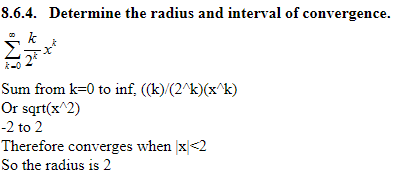8.6.4. Determine the radius and interval of convergence.k-0 to inf,(a)2)Or sqrt(x 2)-2to 2Therefore converges when x2So the radius is 2

Question

did I do this right?

8.6.4.   Determine the radius and interval of convergence.

Sum from k=0 to inf, ((k)/(2^k)(x^k)

Or sqrt(x^2)

-2 to 2

Therefore converges when |x|<2

So the radius is 2help_outlineImage Transcriptionclose8.6.4. Determine the radius and interval of convergence. k-0 to inf,(a)2) Or sqrt(x 2) -2to 2 Therefore converges when x2 So the radius is 2 fullscreen
Step 1

Firstly, we find the radius of convergence as shown on board.

Therefore, series is convergent for every x between -2 to 2.

Step 2

At x=-2, series is oscillting and hence not convergent

At x=2, series is divergent as its  sequence of parti...

Want to see the full answer?

See Solution

Want to see this answer and more?

Our solutions are written by experts, many with advanced degrees, and available 24/7

See Solution
Tagged in

Calculus